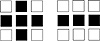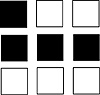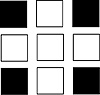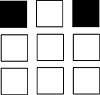# 13.C++ Neural Networks and Fuzzy Logic by Valluru B. Rao M&T Books, IDG Books Worldwide, Inc. ISBN: 1558515526   Pub Date: 06/01/95

#### Binary and Bipolar Inputs

Two types of inputs that are used in neural networks are binary and bipolar inputs. We have already seen examples of binary input. Bipolar inputs have one of two values, 1 and –1. There is clearly a one-to-one mapping or correspondence between them, namely having -1 of bipolar correspond to a 0 of binary. In determining the weight matrix in some situations where binary strings are the inputs, this mapping is used, and when the output appears in bipolar values, the inverse transformation is applied to get the corresponding binary string. A simple example would be that the binary string 1 0 0 1 is mapped onto the bipolar string 1 –1 –1 1; while using the inverse transformation on the bipolar string –1 1 –1 –1, we get the binary string 0 1 0 0.

#### Bias

The use of threshold value can take two forms. One we showed in the example. The activation is compared to the threshold value, and the neuron fires if the threshold value is attained or exceeded. The other way is to add a value to the activation itself, in which case it is called the bias, and then determining the output of the neuron. We will encounter bias and gain later.

### Another Example for the Hopfield Network

You will see in Chapter 12 an application of Kohonen’s feature map for pattern recognition. Here we give an example of pattern association using a Hopfield network. The patterns are some characters. A pattern representing a character becomes an input to a Hopfield network through a bipolar vector. This bipolar vector is generated from the pixel (picture element) grid for the character, with an assignment of a 1 to a black pixel and a -1 to a pixel that is white. A grid size such as 5x7 or higher is usually employed in these approaches. The number of pixels involved will then be 35 or more, which determines the dimension of a bipolar vector for the character pattern.

We will use, for simplicity, a 3x3 grid for character patterns in our example. This means the Hopfield network has 9 neurons in the only layer in the network. Again for simplicity, we use two exemplar patterns, or reference patterns, which are given in Figure 1.5. Consider the pattern on the left as a representation of the character “plus”, +, and the one on the right that of “minus”, - .Figure 1.5  The “plus” pattern and “minus” pattern.

The bipolar vectors that represent the characters in the figure, reading the character pixel patterns row by row, left to right, and top to bottom, with a 1 for black and -1 for white pixels, are C+ = (-1, 1, -1, 1, 1, 1, -1, 1, -1), and C- = (-1, -1, -1, 1, 1, 1, -1, -1, -1). The weight matrix W is:

`       0  0  2 -2 -2 -2  2  0  2       0  0  0  0  0  0  0  2  0       2  0  0 -2 -2 -2  2  0  2       2  0 -2  0  2  2 -2  0 -2 W=    2  0 -2  2  0  2 -2  0 -2       2  0 -2  2  2  0 -2  0 -2       2  0  2 -2 -2 -2  0  0  2       0  2  0  0  0  0  0  0  0       2  0  2 -2 -2 -2  2  0  0 `

The activations with input C+ are given by the vector (-12, 2, -12, 12, 12, 12, -12, 2, -12). With input C-, the activations vector is (-12, -2, -12, 12, 12, 12, -12, -2, -12).

When this Hopfield network uses the threshold function

`              1  if x >= 0 f(x) = {             -1  if x [le] 0 `

the corresponding outputs will be C+ and C-, respectively, showing the stable recall of the exemplar vectors, and establishing an autoassociation for them. When the output vectors are used to construct the corresponding characters, you get the original character patterns.

Let us now input the character pattern in Figure 1.6.Figure 1.6  Corrupted “minus” pattern.

We will call the corresponding bipolar vector A = (1, -1, -1, 1, 1, 1, -1, -1, -1). You get the activation vector (-12, -2, -8, 4, 4, 4, -8, -2, -8) giving the output vector, C- = (-1, -1, -1, 1, 1, 1, -1, -1, -1). In other words, the character -, corrupted slightly, is recalled as the character - by the Hopfield network. The intended pattern is recognized.

We now input a bipolar vector that is different from the vectors corresponding to the exemplars, and see whether the network can store the corresponding pattern. The vector we choose is B = (1, -1, 1, -1, -1, -1, 1, -1, 1). The corresponding neuron activations are given by the vector (12, -2, 12, -4, -4, -4, 12, -2, 12) which causes the output to be the vector (1, -1, 1, -1, -1, -1, 1, -1, 1), same as B. An additional pattern, which is a 3x3 grid with only the corner pixels black, as shown in Figure 1.7, is also recalled since it is autoassociated, by this Hopfield network.Figure 1.7  Pattern result.

If we omit part of the pattern in Figure 1.7, leaving only the top corners black, as in Figure 1.8, we get the bipolar vector D = (1, -1, 1, -1, -1, -1, -1, -1, -1). You can consider this also as an incomplete or corrupted version of the pattern in Figure 1.7. The network activations turn out to be (4, -2, 4, -4, -4, -4, 8, -2, 8) and give the output (1, -1, 1, -1, -1, -1, 1, -1, 1), which is B.Figure 1.8  A partly lost Pattern of Figure 1.7.

### Summary

In this chapter we introduced a neural network as a collection of processing elements distributed over a finite number of layers and interconnected with positive or negative weights, depending on whether cooperation or competition (or inhibition) is intended. The activation of a neuron is basically a weighted sum of its inputs. A threshold function determines the output of the network. There may be layers of neurons in between the input layer and the output layer, and some such middle layers are referred to as hidden layers, others by names such as Grossberg or Kohonen layers, named after the researchers Stephen Grossberg and Teuvo Kohonen, who proposed them and their function. Modification of the weights is the process of training the network, and a network subject to this process is said to be learning during that phase of the operation of the network. In some network operations, a feedback operation is used in which the current output is treated as modified input to the same network.

You have seen a couple of examples of a Hopfield network, one of them for pattern recognition.

Neural networks can be used for problems that can’t be solved with a known formula and for problems with incomplete or noisy data. Neural networks seem to have the capacity to recognize patterns in the data presented to it, and are thus useful in many types of pattern recognition problems.C++ Neural Networks and Fuzzy Logic
ISBN: 1558515526
EAN: 2147483647
Year: 1995
Pages: 139

Similar book on Amazon